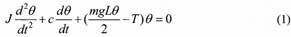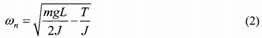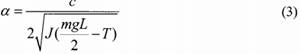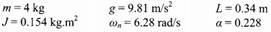### Create an Account

Already have account?

### Forgot Your Password ?

Home / Questions / A computer simulation of the physiological human knee jerk reflex has been developed by Hu...

# A computer simulation of the physiological human knee jerk reflex has been developed by Huang 1994 A strong tap on the patellar ligament of the leg elicits a knee jerk reflex which follows closely

A computer simulation of the physiological human knee jerk reflex has been developed by Huang (1994). A strong tap on the patellar ligament of the leg elicits a knee jerk reflex, which follows closely the oscillations of the pendulum. The jerk of the patellar tendon stretches the muscle that sends a barrage of neural impulses to the spinal cord. The reflex signal passes back to the quadriceps muscle via the alpha motor neuron to produce a sudden contraction and forces the leg to move forward with a jerk. As the muscle relaxes, the leg system acts as a damped compound pendulum, swinging back and forth for a few oscillations. Eventually the leg returns to the normal position.

In his analysis, Huang assumed that the extensor and flexor muscles are identical and opposite in action. The numbers of primary and secondary nerve endings are considered equal, and the nervous signals are instantaneous when compared to the system&#39;s response. Small deflection angles are considered with constant damping coefficient within the range. Based on the equation of the pendulum, and for small oscillations, Huang developed a second order differential equation:that describes the angular position, 0, of the leg during the knee jerk reflex, where m is the mass of the leg, g is the gravitational acceleration constant, L is the length of the leg, J is the moment of inertia of the leg, and T is the gain produced by the isometric torque of the muscle. The natural frequency, ωn, of the system is calculated by:and the damping factor, a, is given by:The values of ωn, and a are obtained experimentally. Solve the above equations with the following values of m, g, L, J, co, and ωn, and plot the time profile of the angular position of the leg during the jerk reflex.Use the following initial conditions θ(0) = 0 rad and dθ(0)/dt = 2πrad/s for the solution of the differential equation (1). HINTS: Use the MATLAB solve command for the algebraic equations and the dsolve command for the differential equation.

Jul 23 2020 View more View Less

#### Answer (Solved)Subscribe To Get Solution